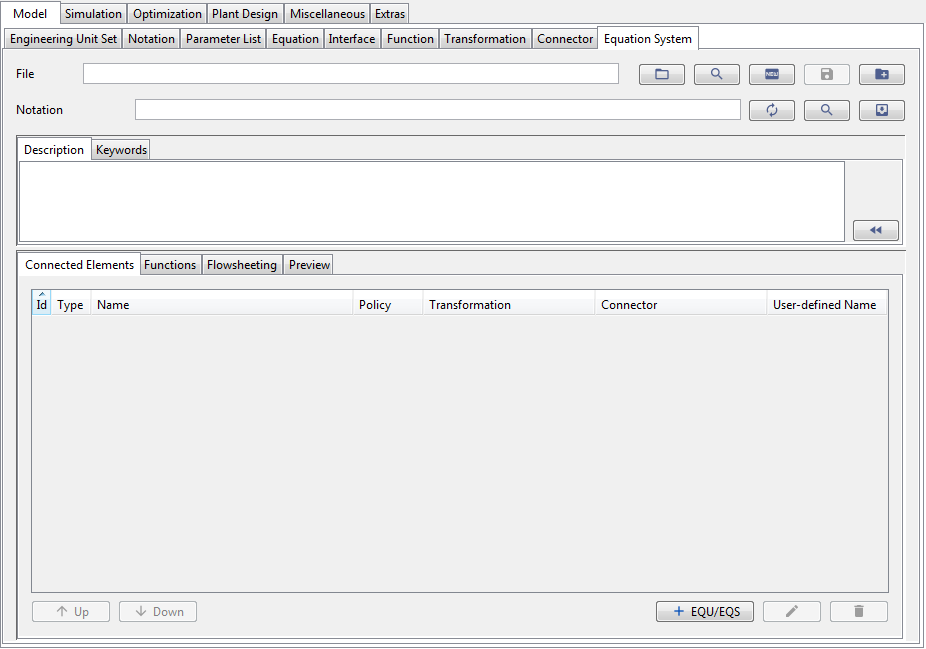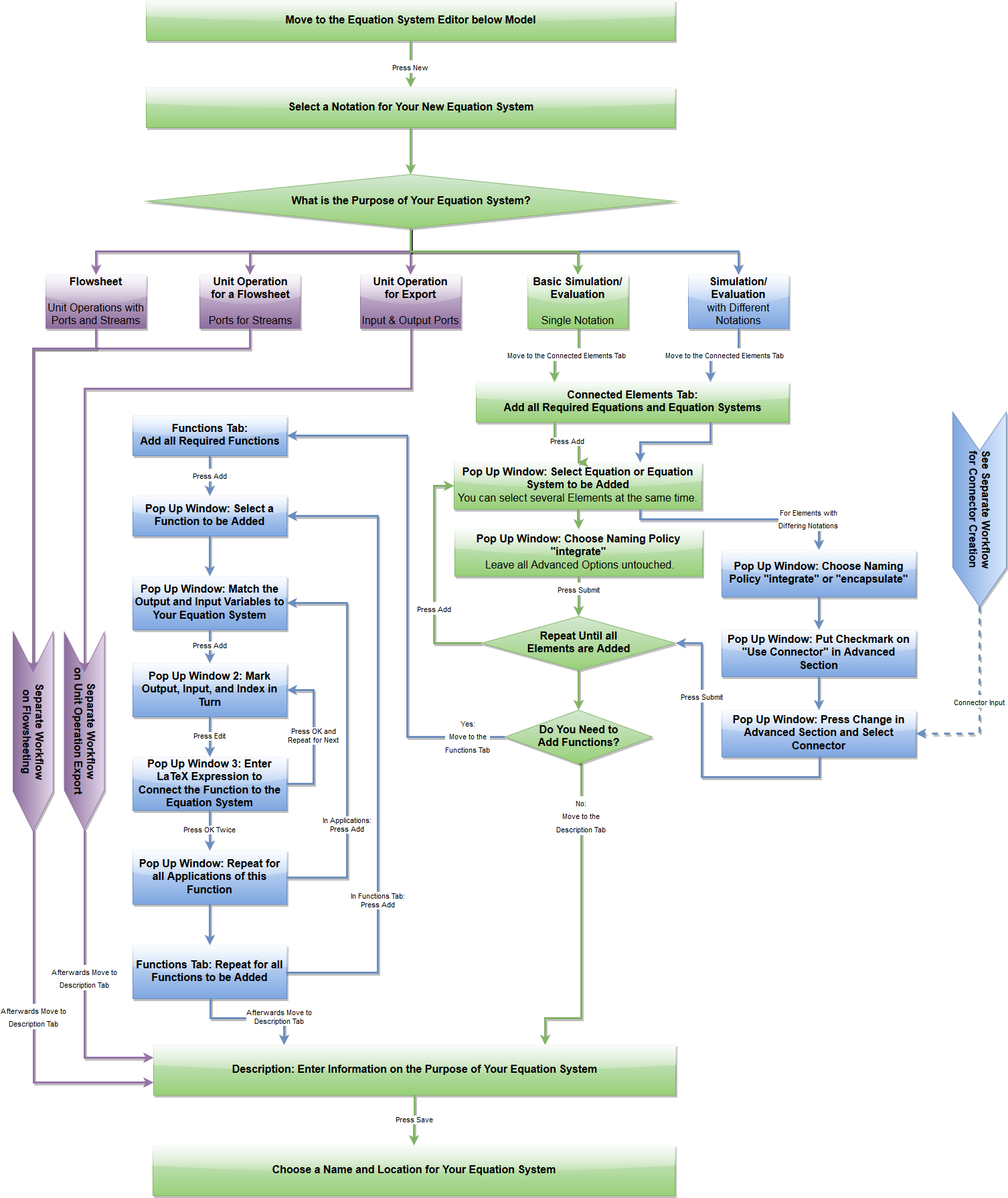# The MOSAICmodeling Workflow – Equation System

### Setting Up an Equation System Inside MOSAICmodeling

To formulate an Equation System inside MOSAICmodeling you need to have created at least one Notation and one Equation. In addition, models may consist of a collection of connected equation systems with different Notations, Functions with Parameter Lists, and may form whole flowsheets with Ports and Streams. These hierarchical levels are sketched in the following figure (‘M’ stands for MOSAICmodeling):In order to set up systems as complex as the one sketched above a number of structures needs to be introduced:

• Notation specifying the nomenclature of an Equation or Equation System
• Equations forming equation (sub-)systems
• Functions calling external or internal calculations
• Connectors connecting Equations or Equation Systems with different Notations
• Ports and Streams providing flowsheeting capabilities and combining unit operations with different Notations

All of these hierarchical levels can be created and connected with the Equation System editor shown in Fig. 1.Figure 1: The Equation System editor of MOSAICmodeling.

The according workflow to navigate the Equation System editor of MOSAICmodeling is given below: